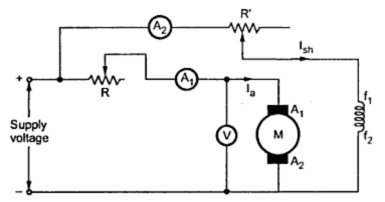Swinburne's Test or No Load Test

This is indirect method of testing d.c. motors in which flux remains practically constant i.e. specially in case of shunt and compound motors. Without actually loading the motor the losses and hence efficiency at different loads can be found out.
The motor is run on no load at its rated voltage. At the starting some resistance is connected in series with the armature which is cut when motor attains sufficient speed.
Now the speed of the motor is adjusted to the rated speed with the help of shunt field rheostat as shown in the Fig. 1.Fig. 1 Swinburnes's
The no load armature current Ia is measured by ammeter A1 whereas the shunt current is measured by ammeter A2.
If V is the supply voltage then motor input at no load will be,
There will be Cu loss in the field winding which will be given as,
Let Ra be the resistance of armature,
Thus the stary losses which includes iron, friction and windage losses can be obtained as,
Stary lossses = Input at no load - Field copper losses - No load armature copper losses
In the field and armature windings there will be copper loss due to flow of current which will increase the temperature of the field and armature winding when the motor is loaded. This increase in temperature will affect their resistance.
Thus the new value of field resistance R'sh and that of armature R'a can be found by considering that rise in temperature as about 40 oC.
If α1 = Resistance temperature coefficient of copper at room temperature
At room temperature the shunt field winding resistance will be,
Rsh = V/ Ish
Now shunt winding current, I'sh = V/R'sh

Now if we want to find the efficiency of the motor at say 1/4 th full load. It can be calculated as follows,
Let      IF.L. = Full load current of motor
WF = Field copper loss
W = Stary losses
Load current at 1/4 th full load =   IF.L./4
...     Motor input at 1/4 th full load = V x ( IF.L./4) watts.
Armature current at 1/4 th full load, I'a  = ( IF.L./4) - I'sh
This is the efficiency of motor when the load on motor is 1/4 th of full load which can be found without loading the motor. The efficiencies at other loads can be calculated similarly.
1. Since constant losses are known, the efficiency can be estimated at any load.
2. The method is convenient and economical as less power is required for testing even a large motor i.e. only no load power is to be supplied.
3. The motor is not required to be loaded i.e. only test to be carried out is the no load test.